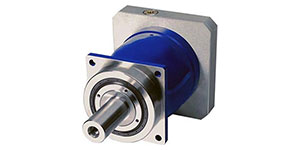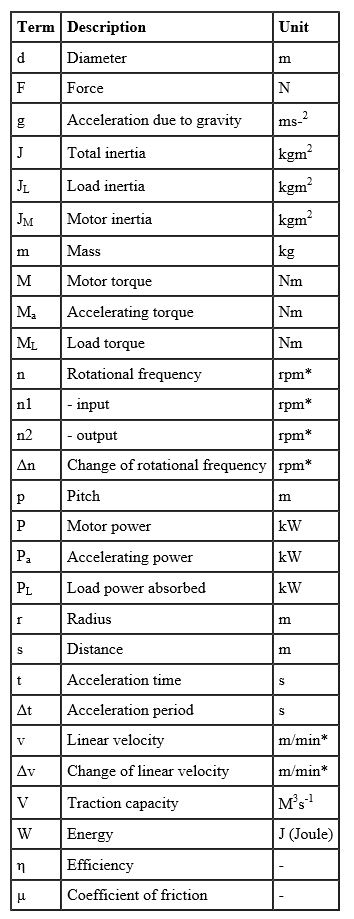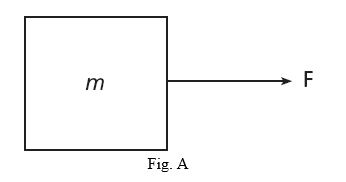## Mechanical Formulas

Vogel 4/17/2018Vogel's guide to mechanical formulas.Note: For practical convenience, some of the units in the formulae following are not S1 units; for example, rotational frequency is commonly measured in revolutions per minute, although the S1 unit is revolutions per second. In these servo drive formulae, the terms used are as tabulated above.Those which are in non-S1 units are marked *.

Linear MotionConsider a body mass m acted upon by a single force F, Fig A. The body accelerates in the direction in which the force is acting, at a rate given by:

A = F/m

After a time t has elapsed, the body has achieved a velocity v, where:

v = u + at

(u is the initial velocity, before the force F was applied. If the body was initially at rest, u is zero)

The distance, s, travelled by the body during time t is

s = ut + at2/2

Distance and velocity are related by the following equation, derived from the two previous ones:

v2 - u2 = 2as

The work done by the force in accelerating the body is the product of force and distance:

W = Fs

The kinetic energy of the body, ie the energy which it possesses by virtue of its motion, is the product of its mass and the square of its velocity:

Ek = mv2/2

Furthermore, since energy is conserved, the work done by the force is equal to the change in the body's kinetic energy (neglecting losses):

W = m(v2 - u2)/2

Power is the rate at which work is done, therefore it is the product of force and velocity:

P = Fv